By Topic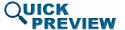• Abstract
• Authors
• Figures
• Multimedia
• References
• Cited By
• Keywords
SECTION I

## INTRODUCTION

Dynamic spectrum allocation is becoming more prevalent in practice, requiring frequency-agile transmitters with amplifiers capable of high-efficiency operation at multiple operating frequencies and power levels based on system needs and limitations. This focus session paper presents a simplex algorithm that is expected to be useful in the real-time fast reconfiguration of power amplifier circuits for wireless communications and radar as they reconfigure between frequencies.

Circuit optimization algorithms originally intended for computer-aided design (CAD) applications can be used for real-time optimization. Bandler, Charalambous, and Steer describe multiple algorithms useful for generic CAD circuit optimization, including simplex (used in this paper), pattern, and gradient searches   . In real-time reconfigurable amplifiers, adaptive amplifier output matching networks are needed. Tunable matching networks using micro-electrical mechanical systems (MEMS) devices are discussed by Lu and Vaka-Heikkila , . Real-time impedance matching is discussed by Deve and Sun    . Qiao demonstrates a reconfigurable low-power communication amplifier using a genetic tuning algorithm , and Baylis, Martin, and Fellows demonstrate unconstrained and constrained optimizations using a gradient-based approach   . This paper demonstrates the use of simplex in amplifier load-impedance optimizations. To our knowledge, this paper represents the first direct application of simplex optimization to optimize in the load-reflection coefficient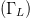$(\Gamma_{L})$ plane.

SECTION II

## SIMPLEX CIRCUIT OPTIMIZATION APPROACH

The simplex method originally presented by Nelder and Mead  and discussed by Bandler  has been applied to our work presented in this paper. In our algorithm, the search is performed in the Smith Chart, which is the complex plane of the load reflection coefficient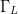$\Gamma_{L}$. Figure 1 shows how the search unfolds. The first point of the simplex is established at the designated starting point, shown as point A. An additional point is then measured in each coordinate direction of the search space, separated from the initial point by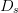$D_{s}$ (points B and C). The “simplex” is the polygon connecting each of the evaluation points. In two dimensions, the initial simplex is a right triangle.

Fig. 1: Simplex construction for the search. The initial simplex consists of points A, B, and C. The optimization plane is the smith chart (the complex plane of$\Gamma_{L}$).

Power-added efficiency (PAE) is used as the optimization criterion for maximization, and is first evaluated at each point of the initial simplex (points A, B, and C). The value of$\Gamma_{L}$ in the simplex possessing the lowest PAE (the worst value of the criterion) is then replaced by a new point .

Figure 1 also illustrates how the replacement simplex point is selected in the case where point A has the lowest (worst) PAE of the original simplex. In this case, PAE is next measured at point 1, the reflection of point A about the centroid of the line connecting the two remaining simplex points B and C . If point 1 has a greater (better) PAE value than both B and C, an additional point is measured at a factor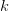$k$ times the initial distance from the line between the other two points (point 2). The PAE at point 2 is then compared with the PAE at point 1. If the PAE at point 2 is greater than the PAE at point 1, then the next simplex consists of points B, C, and 2. This is known as expansion of the simplex. If the PAE at point 2 is lower than at point 1, but point 1 has higher PAE than both B and C, the simplex operations resume using the simplex consisting of points B, C, and 1. If the PAE at point 1 is greater than at point A, but less than the PAE at both B and C, then the new simplex consists of points B, C, and 4 (point 4 is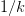$1/k$ times the distance between the centroid and point 1). If point 1 has lower (worse) PAE than the original worst simplex point A, the new simplex point is placed on the original side of the mirroring line at a distance$1/k$ between this line and the original worst point A (this is point 3 in Fig. 2). Simplex operations are re-performed using the simplex consisting of points B, C, and 3. The search ends when the distance from the centroid to the new simplex point falls below a prespecified resolution distance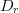$D_{r}$. The highest-PAE point of the final simplex is chosen as the optimum.

SECTION III

## SIMULATION RESULTS

Simulations were performed using a Modelithics model for a Qorvo TGF2960 high electron mobility transistor (HEMT). For the simulation tests, the algorithms were coded in MATLAB, and MATLAB controls simulations of Keysight Technologies' Advanced Design System software. A bias setting of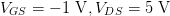$V_{GS}=-1\ \text{V}, V_{DS}=5\ \text{V}$ and input power of 10 dBm were used, with a CDMA2K waveform as the excitation.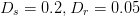$D_{s}=0.2, D_{r}=0.05$, and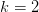$k=2$ were used. Figure 2 shows the simplex search results in the Smith Chart for a starting point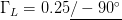$\Gamma_{L}=0.25\underline{/-90^{\circ}}$, An optimum PAE value of 47.64% is obtained at the optimum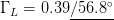$\Gamma_{L}=0.39\underline{/56.8^{\circ}}$ with only 16 measured points. Figure 3 shows that a similar optimum (PAE = 47.64% at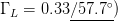$\Gamma_{L}=0.33\underline{/57.7^{\circ}})$ was reached for a starting condition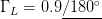$\Gamma_{L}=0.9\underline{/180^{\circ}}$ using 27 measured points. Figure 4 shows a traditional load-pull for PAE, and it can be seen that the efficiency and endpoint values of the searches shown in Figs. 2 and 3 match the optimum from traditional load pull. (PAE = 47.49% at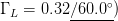$\Gamma_{L}=0.32\underline{/60.0^{\circ}})$.

Fig. 2: Smith chart view of simulated search results starting from$\Gamma_{L}=0.25\underline{/-90^{\circ}}$. 18 measured points were required.

Table I shows the simulation results taken from multiple starting values of$\Gamma_{L}$. The mean end value of PAE is 47.66% with standard deviation of only 0.021 %. The mean number of measurements required for the search for this sample is 18.60 measurements with a sample standard deviation of 5.22.

Fig. 3: Smith chart view of simulated search results starting from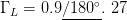$\Gamma_{L}=0.9\underline{/180^{\circ}}.\ 27$ measured points were required.
Fig. 4: Traditional tgf2960 PAE load-pull simulation results. The optimum PAE = 47.49% at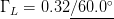$\Gamma_{L}=0.32\underline{/60.0^{\circ}}$
SECTION IV

## MEASUREMENT RESULTS

The algorithm was then measurement tested on a Microwave Technology MWT-173 GaAs MESFET, using a chirp waveform as the excitation. The device is different than the simulation device to provide diversity of situations for algorithm demonstration.$D_{s}=0.2, D_{r}=0.05$, and$k=2$ were used. Figure 5 shows the results for the starting point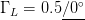$\Gamma_{L}= 0.5\underline{/0^{\circ}}$ (PAE = 32.63% at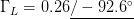$\Gamma_{L}=0.26\underline{/-92.6^{\circ}}$). Figure 6 shows the results for the starting point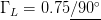$\Gamma_{L}=0.75\underline{/90^{\circ}}$ (PAE = 32.52% at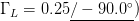$\Gamma_{L}=0.25\underline{/-90.0^{\circ}})$. Figure 7 shows the traditional load-pull results, again showing good correspondence between the endpoints of the measurement search algorithm and the optimum as chosen by a traditional load-pull (PAE = 32.76% at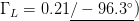$\Gamma_{L}=0.21\underline{/-96.3^{\circ}})$. Table II shows measurement results for multiple starting values of$\Gamma_{L}$.

Table II: Measurement results

The mean end PAE value is 32.63% with a very small sample standard deviation of 0.083%. This shows excellent convergence between the different starting points. In addition, the mean number of measurements for this sample is 19.6, with sample standard deviation of 6.66 measurements.

Fig. 5: Smith chart view of measured search results starting from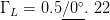$\Gamma_{L}=0.5\underline{/0^{\circ}}.\ 22$ measured points were required.
Fig. 6: Smith chart view of measured search results starting from$\Gamma_{L}=0.75\underline{/90^{\circ}}$. 25 measured points were required.
Fig. 7: Traditional MWT-173 PAE load-pull measurement results. The optimum PAE = 32.76% at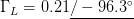$\Gamma_{L}=0.21\underline{/-96.3^{\circ}}$
SECTION V

## CONCLUSIONS

A simplex algorithm for fast load-pull search in the Smith Chart has demonstrated in simulation and measurement. The algorithm has been designed for intended application in a fast, reconfigurable amplifier for use in a frequency-agile transmitter. Ongoing work that cannot be included in this paper due to space limitations, includes constrained optimization, which allows the optimum PAE meeting adjacent-channel power ratio requirements to be quickly found. In addition, ongoing work shows that the simplex method performs very well in comparison to gradient and pattern load-pull searches.

### Acknowldegments

This work has been funded by the National Science Foundation (Grant Number ECCS-1343316). The authors wish to thank Lawrence Cohen of the Naval Research Laboratory for helpful collaboration in this work. In addition, the authors gratefully acknowledge the support of Keysight Technologies in providing cost-free loan of the Advanced Design System software, and Modelithics for donation of simulation model libraries through the Modelithics University Program.

## Footnotes

No Data Available

None

## Corrections

None

This paper appears in:
2016 IEEE MTT-S International Microwave Symposium (IMS)
Conference Date(s):
22-27 May 2016
Conference Location:
San Francisco, CA
On page(s):
1 - 4
E-ISBN:
No Data Available
Print ISBN:
New-2005_Electronic_978-1-5090-0698-4
INSPEC Accession Number:
16213811
Digital Object Identifier:
10.1109/MWSYM.2016.7540376
Date of Current Version:
No Data Available
Date of Original Publication:
No Data Available